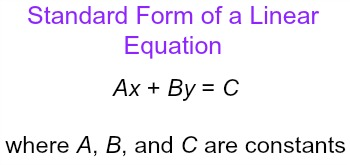# How do you write 3x+9=7/2y in standard form?

Jul 24, 2018

$6 x - 7 y = - 18$

#### Explanation:Let's make it in this form.

First, subtract $\textcolor{b l u e}{\frac{7}{2}}$ from both sides:
$3 x - \frac{7}{2} y + 9 = 0$

Subtract $\textcolor{b l u e}{9}$ from both sides:
$3 x - \frac{7}{2} y = - 9$

In standard form, all the terms also have to be integers $\left(- 3 , - 2 , - 1 , 0 , 1 , 2 , 3. . .\right)$

So multiply both sides by $2$:
$2 \cdot \left(3 x - \frac{7}{2} y\right) = - 9 \cdot 2$

$6 x - 7 y = - 18$

This is now in standard linear form, $A x + B y = C$.

Hope this helps!